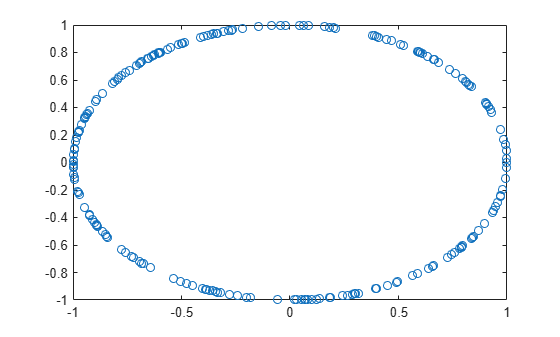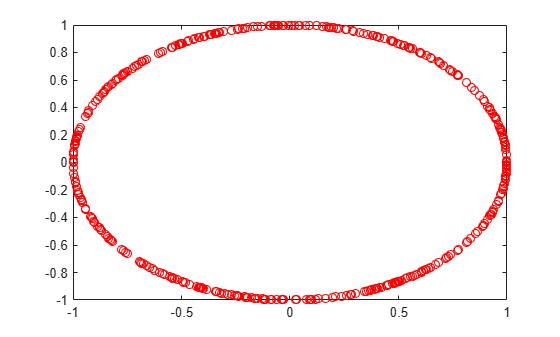# ltePSSCHDRS

PSSCH demodulation reference signal

## Syntax

``````[seq,info] = ltePSSCHDRS(ue)``````

## Description

example

``````[seq,info] = ltePSSCHDRS(ue)``` returns a complex column vector sequence containing PSSCH demodulation reference signal (DM-RS) values and an associated information structure for the specified UE settings structure. For more information, see PSSCH Demodulation Reference Signal Processing.```

## Examples

collapse all

Generate a PSSCH DM-RS sequence associated with both DM-RS SC-FDMA symbols in a subframe. Plot the constellation of the sequence.

Create a user equipment settings structure.

```ue = []; ue.NSAID = 34; ue.NSubframePSSCH = 5; ue.PRBSet = (1:10)';```

Generate a PSSCH DM-RS sequence. Plot the constellation.

```[psschDrsSeq,info] = ltePSSCHDRS(ue); plot(psschDrsSeq,'o')```Generate a PSSCH DM-RS sequence for V2X using the format 1 SCI PSCCH CRC.

Create a user equipment settings structure.

``` ue = []; ue.SidelinkMode = 'V2X'; ue.PRBSet = (1:10)'; ue.NSLRB = 50;```

Generate the format 1 SCI PSCCH CRC and assign it to the UE V2X scrambling identity.

``` sciinfo = lteSCIInfo(ue); scibits = ones(1,sciinfo.Format1); [cw,crc] = lteSCIEncode(ue,scibits); ue.NXID = crc;```

Generate a PSSCH DM-RS sequence. Plot the constellation.

```[psschDrsSeq,info] = ltePSSCHDRS(ue); plot(psschDrsSeq,'or')```## Input Arguments

collapse all

User equipment settings, specified as a parameter structure containing these fields:

Sidelink mode, specified as `'D2D'` or `'V2X'`.

Data Types: `char` | `string`

Sidelink group destination identity, specified as an integer in the interval [0, 255].

This field is the lower eight bits of the full 24-bit ProSe Layer-2 group destination ID. This field and the `NSubframePSSCH` field control the value of the scrambling sequence at the start of each subframe. This field is required only for D2D sidelink.

Data Types: `double`

V2X scrambling identity, specified as an integer scalar. `NXID` is the 16 bit CRC associated with the PSCCH SCI grant. It is only required for V2X sidelink.

Data Types: `double`

PSSCH subframe number in the PSSCH subframe pool, specified as an integer scalar. (${n}_{\text{ssf}}^{\text{PSSCH}}$)

`NSubframePSSCH` and `NSAID` control the values of the scrambling sequence. It is only required for D2D sidelink.

Data Types: `double`

Zero-based physical resource block (PRB) indices, specified as an integer column vector or a two-column integer matrix.

The PSSCH is intended to be transmitted in the same PRB in each slot of a subframe. Therefore, specifying `PRBSet` as a single column of PRB indices is recommended. However, for a nonstandard slot-hopping PRB allocation, `PRBSet` can be specified as a two-column matrix of indices corresponding to slot-wise resource allocations for PSSCH.

Data Types: `double`

Data Types: `struct`

## Output Arguments

collapse all

PSSCH DM-RS values, returned as a N × 12 × NPRB-by-1 column vector. For more information, see PSSCH Demodulation Reference Signal Processing.

PSSCH DM-RS information about the intermediate variables used to create the DM-RS, returned as a parameter structure containing these fields:

Reference signal cyclic shift for each slot, returned as a two-column vector. (α)

`Alpha` is proportional to `NCS`, where $\alpha =\frac{2\pi {n}_{cs,\lambda }}{12}$.

Base sequence group number for each slot, returned as a two-column vector. (u)

Base sequence number for each slot, returned as a two-column vector. (v)

Root Zadoff-Chu sequence index for each slot, returned as a two-column vector. (q)

Cyclic shift values for each slot, returned as a two-column vector. (${n}_{\text{cs,}\lambda }$)

Zadoff-Chu sequence length, returned as an integer. (${N}_{ZC}^{RS}$)

Orthogonal cover value for each slot, returned as a matrix. ($\overline{w}$)

Data Types: `struct`

collapse all

### PSSCH Demodulation Reference Signal Processing

The PSSCH demodulation reference signal (DM-RS) sequence is transmitted alongside the `ltePSSCH` values using the two SC-FDMA symbols allocated to DM-RS in a PSSCH subframe. The output vector is the repetition of a 12-element sequence and specified in TS 36.211, Section 9.8. The vector is mapped onto the 12 DM-RS SC-FDMA symbol subcarriers in each subframe slot for each PSSCH physical resource block (PRB) transmission on antenna port 1000.

The output PSSCH DM-RS sequence is the concatenation of the two sequences to be mapped onto the DM-RS SC-FDMA symbol subcarriers in each subframe slot carrying a `ltePSSCH` transmission. Its length is N × 12 × NPRB, where NPRB is the number of PRBs associated with the PSSCH. For D2D sidelink, there is one DM-RS symbol per slot and therefore N=2, and for V2X sidelink, there are two symbols per slot and N=4.

### PSSCH Demodulation Reference Signal Indexing

Use the `ltePSSCHDRSIndices` indexing function and the corresponding `ltePSCCHDRS` sequence function to populate the resource grid for any PSSCH subframe. The PSSCH DM-RS is transmitted in the available SC-FDMA symbols in a PSSCH subframe, using a single layer on antenna port 1000.

The indices are ordered as the PSSCH DM-RS QPSK modulation symbols should be, applying frequency-first mapping. One-based linear indexing is the default return format, but alternative indexing formats can also be generated.

The resource elements in the last SC-FDMA symbol within a subframe are counted in the mapping process but should not be transmitted. The sidelink-specific SC-FDMA modulation creates the last symbol, which serves as a guard symbol.

For D2D sidelink, when indexing is zero-based, the SC-FDMA symbol indices used are {3,10} for normal cyclic prefix and {2,8} for extended cyclic prefix. The same symbols are used by the `ltePUSCHDRSIndices` function. For V2X sidelink, there are four DM-RS SC-FDMA symbols with indices {2,5,8,11} for normal cyclic prefix only.

Note

The indicated symbol indices are based on TS 36.211, Section 9.8. However, to align with the LTE Toolbox™ subframe orientation, these indices are expanded from symbol index per slot to symbol index per subframe.

For more information on mapping symbols to the resource element grid, see Resource Grid Indexing.

 3GPP TS 36.211. “Evolved Universal Terrestrial Radio Access (E-UTRA); Physical Channels and Modulation.” 3rd Generation Partnership Project; Technical Specification Group Radio Access Network. URL: https://www.3gpp.org.